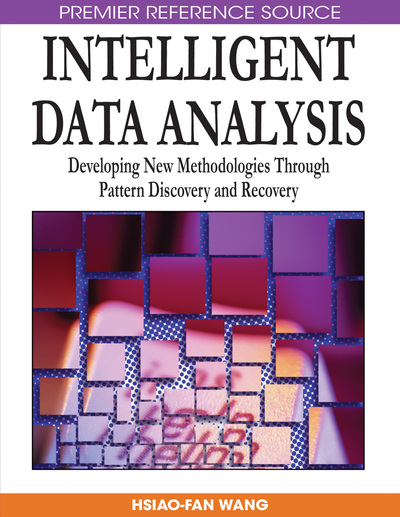# Design and Development of Knowledge Bases for Forward and Reverse Mappings of TIG Welding Process

J. P. Ganjigatti (Siddaganga Institute of Technology, Tumkur, India) and Dilip Kumar Pratihar (Indian Institute of Technology, Kharagpur, India)
Copyright: © 2009 |Pages: 16
DOI: 10.4018/978-1-59904-982-3.ch011
OnDemand PDF Download:
Available
\$37.50
No Current Special Offers

## Abstract

In this chapter, an attempt has been made to design suitable knowledge bases (KBs) for carrying out forward and reverse mappings of a Tungsten inert gas (TIG) welding process. In forward mapping, the outputs (also known as the responses) are expressed as the functions of the input variables (also called the factors), whereas in reverse mapping, the factors are represented as the functions of the responses. Both the forward as well as reverse mappings are required to conduct, for an effective online control of a process. Conventional statistical regression analysis is able to carry out the forward mapping efficiently but it may not be always able to solve the problem of reverse mapping. It is a novel attempt to conduct the forward and reverse mappings of a TIG welding process using fuzzy logic (FL)-based approaches and these are found to solve the said problem efficiently.
Chapter Preview
Top

## Introduction

We have a natural quest to know the input-output relationships of a process. To control the process efficiently, it might be required to know the said relationships beforehand. Forward mapping aims to determine the outputs as the functions of the inputs, whereas the inputs are expressed as the functions of the outputs in reverse mapping. Both the forward as well as reverse mappings of a process are to be carried out, to automate the same. It may be difficult to determine the input-output relationships for most of the processes mathematically, due to inherent complexity of their physics. Realizing this, several attempts were made by various investigators to express the said relationships through conventional statistical regression analysis. It is carried out based on the data collected experimentally, which may not be precise in nature. Forward mapping can be done easily but reverse mapping may sometimes offer difficulty. It happens, when the transformation matrix relating the outputs with the inputs becomes singular. In such cases, particularly when the transformation matrix becomes non-square, some investigators tried to determine the pseudo-inverse of the matrix. However, it may be far from being the true inverse of the transformation matrix and as a result of which, the deviation in predictions of the inputs for a set of desired outputs could be more. To ensure a square transformation matrix between the outputs and inputs of a process, the number of outputs should be equal to that of inputs. In order to make the reverse mapping possible, the interaction terms of linear regression model are to be neglected. It is important to mention that reverse mapping cannot be implemented accurately for the nonlinear regression model. Forward mapping can be carried out through the linear and non-linear regression models, if the experimental data are collected as per some standard two-level and three-level designs of experiments, respectively. On the other hand, reverse mapping can be implemented using the conventional linear regression analysis involving main factors only, for a process having equal number of inputs and outputs. The above discussion has been summarized in Table 1.

Table 1.
Requirements for forward and reverse mappings
 Mappings Requirements Forward • Linear regression analysis based on two-level designs of experiments like full-factorial, fractional factorial.     • Non-linear regression analysis based on three-level designs of experiments, namely central composite design, Box-Behnken design, and so on. Reverse • Linear regression analysis involving main factors only for a process having the same number of inputs and outputs.

In conventional statistical regression analysis, only one output parameter is tackled at a time and consequently, it may not be able to capture the dynamics of the process completely. Soft computing is a family consists of the techniques—Fuzzy Logic (FL), Neural Networks (NN), Genetic Algorithms (GAs), and their different combinations, in which the precision is traded for tractability, robustness, and a low cost solution. It may provide with some feasible solutions to the said problem.

## Complete Chapter List

Search this Book:
Reset